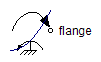Square Root - MapleSim Help

Square Root

Compute the square rootDescription The Square Root (or Sqrt) component computes the element-wise square root of the input $u$. Each input must be nonnegative. The Signal Size parameter assigns the dimension of the input and output connectors.
 Equations ${y}_{j}=\sqrt{{u}_{j}}$

Connections

 Name Description Modelica ID $u$ Real input vector u $y$ Real output vector y

Parameters

 Name Default Units Description Modelica ID Signal Size $1$ Dimension of input and output signals signalSize

 Modelica Standard Library The component described in this topic is from the Modelica Standard Library. To view the original documentation, which includes author and copyright information, click here.